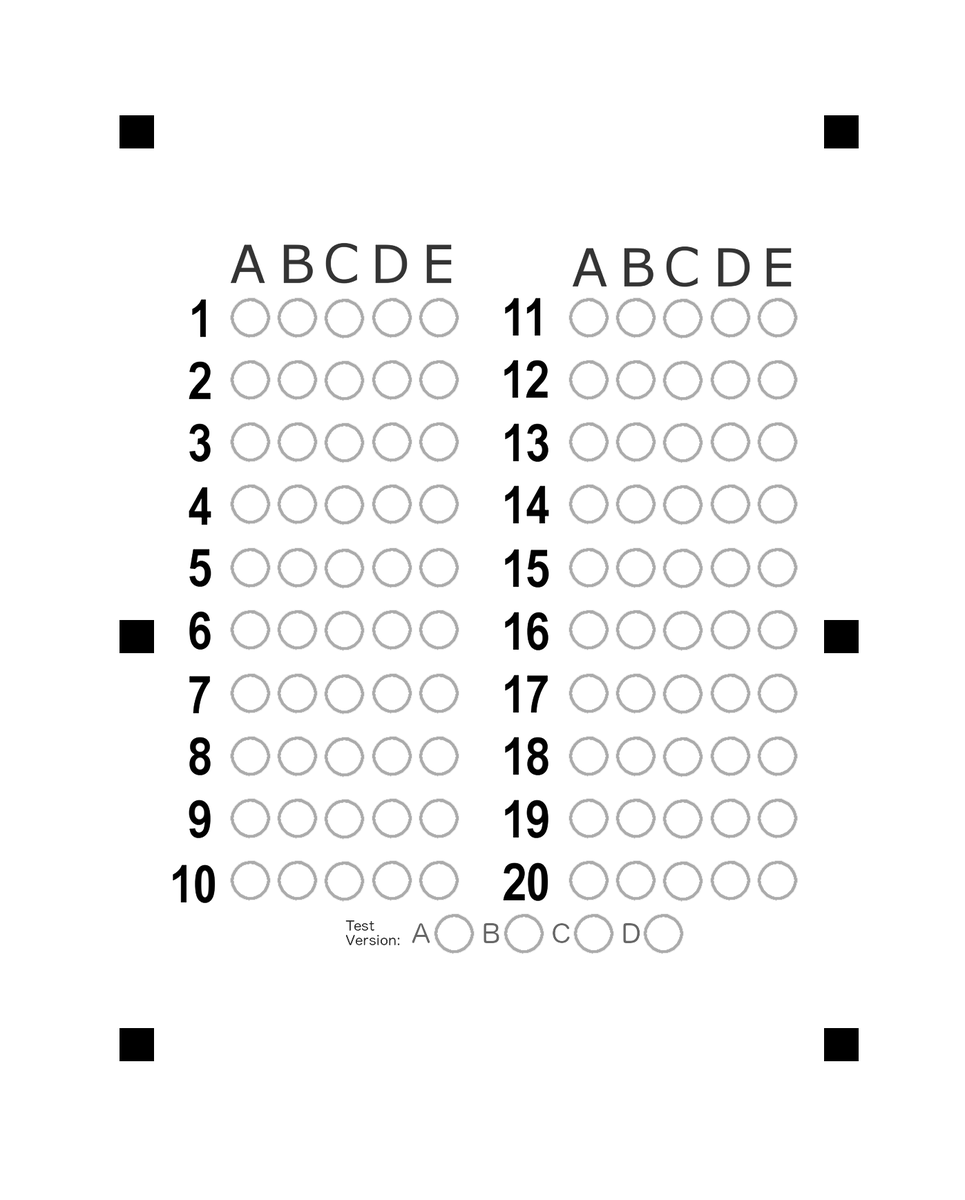# Sunshine CTF 2019 writeup

I attended Sunshine CTF 2019 as a member of zer0pts. We came in the 10th place with 3255 points at the end of the CTF. I regret that I gave up to solve challenges during the competition.

I solved only two scripting challenges. I'm going to write up.

## [Scripting 50 (125 Solves)]TimeWarp

Oh no! A t3mp0ral anoma1y has di5rup7ed the timeline! Y0u'll have to 4nswer the qu3stion5 before we ask them!

`nc tw.sunshinectf.org 4101`

Author: Mesaj2000

This was a numbers game challenge. The server demanded us to guess many numbers in 30 seconds. As I send my guessing number, the server returns the correct number that doesn't change in every attempt. So we could get the n-th correct number at the n-th attempt. I used the python script first, however, it was slow. So I rewrote it to the following simple shell script.

```lines="39"  # the first correct number

while true; do
newx=\$(echo -e "\$lines\n9999" | nc tw.sunshinectf.org 4101 | tail -2 | grep -o -E '^[0-9]+\$')
lines=\$(echo -e "\$lines\n\$newx")
echo \$newx
done
```
```I'm going to give you some numbers between 0 and 999.
Repeat them back to me in 30 seconds or less!
39
Alm0st there!
61
N1ce 0ne! Do it ag4in!

(...snip...)

Keep it up! The anoma1y is subsiding!
326
Icr3dible!
370
Wow! You did it!
As reward for fixing the timestream, here's the flag:
sun{derotser_enilemit_1001130519}```

## [Scripting 250 (47 Solves)]Entry Exam

I heard the Hart Foundation is accepting applications, see if you have what it takes by completing their entry exam.

http://ee.sunshinectf.org

Author: dmaria

The web page destributed a mark sheet as PNG format and took mathematics exams. To get the flag, we needed to solve 20 problems and mark the correct positions, submit a marked sheet in 2 seconds.Pillow is a very powerful python library for image manipulation. I used it to mark. Remaining tasks are easy: get the problems, solve them, and submit a marked sheet.

The flag was `sun{7h3_b357_7h3r3_15_7h3_b357_7h3r3_w45_7h3_b357_7h3r3_3v3r_w1ll_b3}`.

```from PIL import Image, ImageDraw
import requests
import re

R = 25
DX = 68
DY = 90
XOFF = 362
XOFF2 = 852 - XOFF
YOFF = 460

x = XOFF + answer * DX
if problem >= 11:
x += XOFF2
problem -= 10
problem -= 1
y = YOFF + problem * DY

draw.ellipse((x-R, y-R, x+R, y+R), fill=0)

URL = 'http://ee.sunshinectf.org/exam'
s = requests.Session()

r = s.get(URL)
lines = r.text.splitlines()[1:-9]

while True:
problem_num = 1
img = Image.open("scantron.png").convert('L')
draw = ImageDraw.Draw(img)

for i in range(0, len(lines), 7):
ans = str(eval(re.findall('>(.+)<', lines[i]).replace('/', '//')))
hoge = None
for j in range(2, 6):
if ans == re.findall('>(.+)<', lines[i+j]):
hoge = j - 2
break
assert(hoge is not None)

print(lines[i], ans, hoge, lines[i+hoge+2])
check_at(draw, problem_num, hoge)
problem_num += 1

img.save('tmp.png')
image = open('tmp.png', 'rb')
files = {'file': ('tmp.png', image, 'image/png')}
r = s.post(URL, files=files)
lines = r.text.splitlines()

print(lines)
if 'Section' not in lines:
print(r.text)
break
lines = lines[1:-9]
```